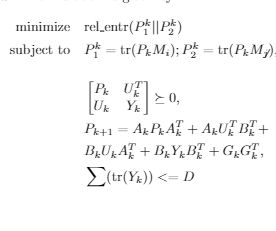# Minimize log(x/y) using cvx

Hi,
I am trying to minimize
log(x/y) where
x = trace(PM); y = trace(PN);
where M, N are symmetric matrices and P is positive definite and x>y.
P is given by
P = AQA’ + AUB’ + BUA’ + BYB’;
where P*Y = U^TU; M = [A(1,1)^2 A(1,1)A(1,2);A(1,1)A(1,2) A(1,2)^2];
N = [A(1,1)^2 0;0 0];
U is the variable.
I have tried with minimizing xlog(x/y) and it minimizes the log(x/y) as well.
so my questions are

1. Is minimizing xlog(x/y) approximates minimizing log(x/y) for x>y>0?
2. Is there a way to approximate a convex function of log(x/y) using some slack variable?I believe you can enter the problem in the image almost “as is”, using CVX’s `rel_entr` function.

As for solving it, use Mosek if available to you, otherwise follow the instructions at CVXQUAD: How to use CVXQUAD's Pade Approximant instead of CVX's unreliable Successive Approximation for GP mode, log, exp, entr, rel_entr, kl_div, log_det, det_rootn, exponential cone. CVXQUAD's Quantum (Matrix) Entropy & Matrix Log related functions

Yes Mark,
The image is what I approximated for log(P_1/P_2). But my question again is minimizing log(P_1/P_2) equal to or approximate to minimizing the rel_entr in the image.

Thanks for the quick response.

It’s different.

I presumed the problem in the image is what you want to solve, and that is a convex optimization problem which can easily be entered in CVX. `log(P_1/P_2)` in which `P1` and `P2` are both optimization variables, is neither convex nor concave, and can’t be entered as such in CVX.

1 Like